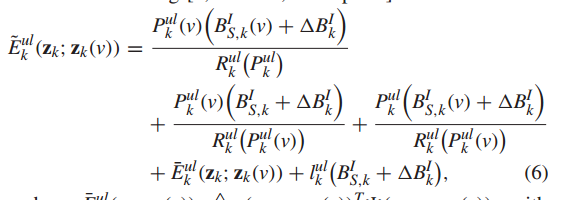# Cannot perform the operation: {concave} ./ {concave}

Dear everyone:-)
I got some question when I tried to used cvx.
My code is followed:
clear;
clear all;
k = 8;
%n = [0,0.1,0.2,0.3,0.4,0.5,0.6,0.7,0.8,0.9,1];
n = 0.3;
r=0.9;%暂时假设
%仿真参数设置;
N0 = -147; Wul = 10000; Wdl = 10000;
Pumax = 100; Pdmax = 1000; Fc = 10^10;%dbm=10lg(P/1mw)
lu = 1.7810^-6; ld = 0.625;
BIk = 1000
ones(1,k); BOk = 1000ones(1,k);
Vk = 2640
BIk; Vs = nVk;
BOs = n
BOk; BIs = nBIk;
%初始化z0 = [Pul,Bls,f,Pdl,PdM,TuS,TdS];
Pul0 = Pumax
rand(1,k); Bls0 = BIs/k; Pdl0 = Pdmaxrand(1,k); PdM0 = Pdmaxrand(1,1);
fk0 = rand(1,k); fs0 = rand(1,1); f0 = [fk0,fs0];

Rul0 = (Wul/k)log2(1+((rPul0)/10^((N0+10log10(Wul/k))-3)));
Rdl0 = (Wdl/k)log2(1+((rPdl0)/10^((N0+10
log10(Wdl/k))-3)));
RdM0 = Wdllog2(1+((rPdM0)/10^((N0+10*log10(Wdl))-3)));

TuS0 = max(Bls0./Rul0); TdS0 = max(BOs./RdM0);

z0 = [Pul0,Bls0,f0,Pdl0,PdM0,TuS0,TdS0];
a = 10^-5; e = 10^-5;

``````%求解最优解
%cvx_solver mosek
cvx_begin
%variable Pul(1,k) Bls(1,k) fk(1,k) fs Pdl(1,k) PdM TuS TdS;
variable Pul(1,k);
variable Bls(1,k);
variable fk(1,k);
variable Pdl(1,k);
variable fs;
variable PdM;
variable TuS;
variable TdS;
f = [fk,fs];
Rul = (Wul/k)*log(1+((r*Pul)/10^((N0+10*log(Wul/k))-3)))/log(2);
Rdl = (Wdl/k)*log(1+((r*Pdl)/10^((N0+10*log(Wdl/k))-3)))/log(2);
RdM = Wdl*log(1+((r*PdM)/10^((N0+10*log(Wdl))-3)))/log(2);

%TuS = max(Bls.*inv_pos(Rul));
%TdS = max(BOs.*inv_pos(RdM));
%TuS = max(Bls./Rul);
%TdS = max(BOs./RdM);
%Tmax = TuS+Vs/(fs*Fc)+TdS+max((BIk - Bls)/Rul+(Vk-Vs)/(fk*Fc)+(BOk-BOs)/Rdl);
z = [Pul,Bls,f,Pdl,PdM,TuS,TdS];
a = square_pos(Bls+inv_pos(Rul));
b = square_pos(Rul0);
c = Bls0.*(Bls-Bls0);
d = 10^((N0+10*log10(Wul/k))-3);
e = 1+(r*Pul0)*inv_pos(d).*square_pos(Rul0).*square_pos(Rul0);
f = c-Rul0/e*(inv_pos(Rul)-inv_pos(Rul0));
g = (a-square_pos(Bls0)-1./b);
gk = 1/2*g-f;
%gk = 1/2*((Bls+1/Rul)^2-Bls0^2-1/Rul0^2)-(Bls0*(Bls-Bls0)-Rul0/((1+(r*Pul0)/10^((N0+10*log10(Wul/k))-3))*Rul0^4)*(1/Rul-1/Rul0));
Edl = ((BOk-BOs).*inv_pos(Rdl)+BOs*inv_pos(RdM))*ld;
E = (z-z0)*diag(z0)*(z-z0)';

a1 = Pul0.*(Bls0+(BIk-pos(Bls)))./Rul;
b1 = Pul0.*(Bls+(BIk-Bls))./Rul0;
c1 = Pul.*(Bls0+(BIk-Bls))./Rul0;
d1 = lu*(Bls+(BIk-Bls));
Eul = a1+b1+c+E+d1;
minimize(sum(Eul+Edl))
%minimize(sum(Pul0.*(Bls0+(BIk-Bls))./Rul+Pul0.*(Bls+(BIk-Bls))./Rul0+Pul.*(Bls0+(BIk-Bls))./Rul0+(z-z0)*diag(z-z0)+lu*(Bls+(BIk-Bls))+((BOk-BOs)./Rdl+BOs./RdM)*ld))
subject to
(BIk - Bls)/Rul+(Vk-Vs)/(fk*Fc)+Vs/(fs*Fc)+(BOk-BOs)/Rdl <= Tmax-TuS-TdS    %约束条件C.1
gk <= TuS    %约束条件C.2
BOs/RdM <= TdS    %约束条件C.3
sum(fk) <= 1      %约束条件C.4
fk >= 0
0 <= fs <= 1
sum(Bls) == BIs   %约束条件C.5
sum(Pdl) <= Pdmax    %约束条件C.6
PdM <= Pdmax
Pul <= Pumax
cvx_end

The error is:
Error using cvx/times (line 173)
``````

Disciplined convex programming error:
Cannot perform the operation: {concave} ./ {concave}

Error in cvx/rdivide (line 19)
z = times( x, y, ‘./’ );

Error in cvx_test (line 65)
a1 = Pul0.*(Bls0+(BIk-pos(Bls)))./Rul;

``````I would like to know if the objective function needs x and y to multiply or divide, how to convert it so that cvx can accept the model?

Can anyone give me some advice? Thank you!
``````

Xiancan Lian

How have you proven that your problem is convex? If it is not convex, there is no possibility of entering it into CVX.

This question will be marked non-convex, unless you are able to show that it is convex.

Thanks Mark L. Stone. I have to make the simulation in a document, the formula in the document has been described as a convex function. My question now is how should I convert my target function into a form that cvx can recognize by using some function like rel_entr() and so on?Simply said. I have two variables x, y. They are vectors, the objective function is x/y. I transform them into a form x/y = entr(x)/(entr(y) - rel_entr(y,x)).
Does it work? Beacause I got the error like this:
Error using cvx/rel_entr (line 71)
Disciplined convex programming error:
Illegal operation: rel_entr( {concave}, {positive constant} ).

Error in entr (line 20)
y = -rel_entr( x, 1 );

Error in cvx_test (line 62)
A1 = entr(Bls)/(entr(Rul)-rel_entr(Rul,Bls));

You didn’t have entr or rel_entr in your first post. The image in your 2nd post is cut off. I don’t know what you are trying to do. What exactly is convex? You have so many different things in your posts that I don’t know what’s what.